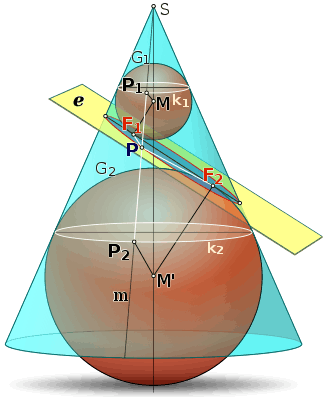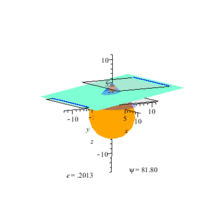# Dandelin spheresDandelin spheres are touching the light blue plane that intersects the cone.

In geometry, the Dandelin spheres are one or two spheres that are tangent both to a plane and to a cone that intersects the plane. The intersection of the cone and the plane is a conic section, and the point at which either sphere touches the plane is a focus of the conic section, so the Dandelin spheres are also sometimes called focal spheres.

The Dandelin spheres were discovered in 1822. They are named in honor of the Belgian mathematician Germinal Pierre Dandelin, though Adolphe Quetelet is sometimes given partial credit as well. The Dandelin spheres can be used to prove at least two important theorems. Both of those theorems were known for centuries before Dandelin, but he made it easier to prove them.

The first theorem is that a closed conic section (i.e. an ellipse) is the locus of points such that the sum of the distances to two fixed points (the foci) is constant. This was known to Ancient Greek mathematicians such as Apollonius of Perga, but the Dandelin spheres facilitate the proof.

The second theorem is that for any conic section, the distance from a fixed point (the focus) is proportional to the distance from a fixed line (the directrix), the constant of proportionality being called the eccentricity. Again, this theorem was known to the Ancient Greeks, such as Pappus of Alexandria, but the Dandelin spheres facilitate the proof.

A conic section has one Dandelin sphere for each focus. In particular, an ellipse has two Dandelin spheres, both touching the same nappe of the cone. A hyperbola has two Dandelin spheres, touching opposite nappes of the cone. A parabola has just one Dandelin sphere.

## Proof that the curve has constant sum of distances to foci

Consider the illustration, depicting a plane intersecting a cone to form an ellipse (the interior of the ellipse is colored light blue). The two Dandelin spheres are shown, one (G1) above the ellipse, and one (G2) below. The intersection of each sphere with the cone is a circle (colored white).

• Each sphere touches the plane at a point, and let us call those two points F1 and F2.
• Let P be a typical point on the ellipse.
• Prove: The sum of distances d(F1, P) + d(F2, P) remain constant as the point P moves along the curve.
• A line passing through P and the vertex S of the cone intersects the two circles at points P1 and P2.
• As P moves along the ellipse, P1 and P2 move along the two circles.
• The distance from F1 to P is the same as the distance from P1 to P, because lines PF1 and PP1 are both tangent to the same sphere (G1).
• Likewise, the distance from F2 to P is the same as the distance from P2 to P, because lines PF2 and PP2 are both tangent to the same sphere (G2).
• Consequently, the sum of distances d(F1, P) + d(F2, P) must be constant as P moves along the curve because the sum of distances d(P1, P) + d(P2, P) also remains constant.
• This follows from the fact that P lies on the straight line from P1 to P2, and the distance from P1 to P2 remains constant.

This proves a result that had been proved in a different manner by Apollonius of Perga.

If (as is often done) one takes the definition of the ellipse to be the locus of points P such that d(F1, P) + d(F2, P) = a constant, then the argument above proves that the intersection of a plane with a cone is indeed an ellipse. That the intersection of the plane with the cone is symmetric about the perpendicular bisector of the line through F1 and F2 may be counterintuitive, but this argument makes it clear.

Adaptations of this argument work for hyperbolas and parabolas as intersections of a plane with a cone. Another adaptation works for an ellipse realized as the intersection of a plane with a right circular cylinder.Dandelin spheres, ellipse, directrices (blue lines).

## Proof of the focus-directrix property

The directrix of a conic section can be found using Dandelin's construction. Each Dandelin sphere intersects the cone at a circle; let both of these circles define their own planes. The intersections of these two parallel planes with the conic section's plane will be two parallel lines; these lines are the directrices of the conic section. However, a parabola has only one Dandelin sphere, and thus has only one directrix.

Using the Dandelin spheres, it can be proved that any conic section is the locus of points for which the distance from a point (focus) is proportional to the distance from the directrix. Ancient Greek mathematicians such as Pappus of Alexandria were aware of this property, but the Dandelin spheres facilitate the proof.

Neither Dandelin nor Quetelet used the Dandelin spheres to prove the focus-directrix property. The first to do so was apparently Pierce Morton in 1829. The focus-directrix property is essential to proving that astronomical objects move along conic sections around the Sun.

1. Taylor, Charles. An Introduction to the Ancient and Modern Geometry of Conics, page 196 ("focal spheres"), pages 204–205 (history of discovery) (Deighton, Bell and co., 1881).
2. Kendig, Keith. Conics, page 86 (proof for ellipse) and page 141 (for hyperbola) (Cambridge University Press, 2005).
3. Heath, Thomas. A History of Greek Mathematics, page 119 (focus-directrix property), page 542 (sum of distances to foci property) (Clarendon Press, 1921).
4. Brannan, A. et al. Geometry, page 19 (Cambridge University Press, 1999).
5. Morton, Pierce. Geometry, Plane, Solid, and Spherical, in Six Books, page 228 (Baldwin and Cradock, 1830).
6. Hyman, Andrew. "A Simple Cartesian Treatment of Planetary Motion", European Journal of Physics, Vol. 14, page 145 (1993).Wikimedia Commons has media related to Dandelin spheres.